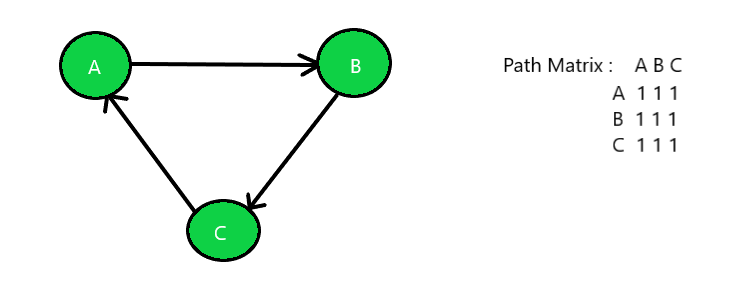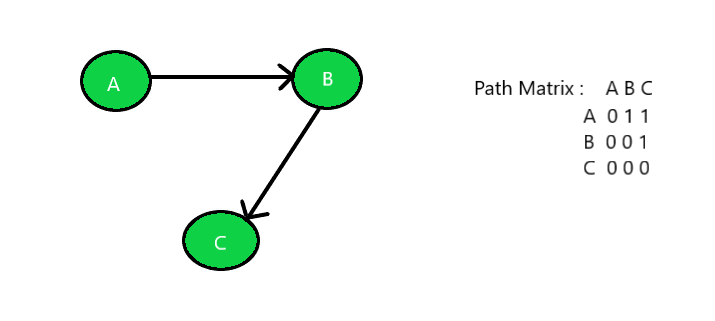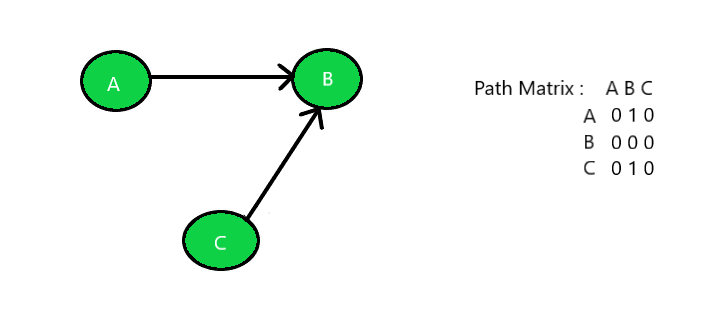# Check if a graph is Strongly, Unilaterally or Weakly connected

Given an unweighted directed graph G as a path matrix, the task is to find out if the graph is Strongly Connected or Unilaterally Connected or Weakly Connected.

Strongly Connected: A graph is said to be strongly connected if every pair of vertices(u, v) in the graph contains a path between each other. In an unweighted directed graph G, every pair of vertices u and v should have a path in each direction between them i.e., bidirectional path. The elements of the path matrix of such a graph will contain all 1’s.

Unilaterally Connected: A graph is said to be unilaterally connected if it contains a directed path from u to v OR a directed path from v to u for every pair of vertices u, v. Hence, at least for any pair of vertices, one vertex should be reachable form the other. Such a path matrix would rather have upper triangle elements containing 1’s OR lower triangle elements containing 1’s.

Weakly Connected: A graph is said to be weakly connected if there doesn’t exist any path between any two pairs of vertices. Hence, if a graph G doesn’t contain a directed path (from u to v or from v to u for every pair of vertices u, v) then it is weakly connected. The elements of such a path matrix of this graph would be random.

Examples:

Input: Below is the given graph with path matrix:Output: Strongly Connected Graph

Input: Below is the given graph with path matrix:Output: Unilaterally Connected Graph

Input: Below is the given graph with path matrix:Output: Weakly Connected Graph

## Recommended: Please try your approach on {IDE} first, before moving on to the solution.

Approach:

1. For the graph to be Strongly Connected, traverse the given path matrix using the approach discussed in this article check whether all the values in the cell are 1 or not. If yes then print “Strongly Connected Graph” else check for the other two graph.
2. For the graph to be Unilaterally Connected, traverse the given path matrix using the approach discussed in this article and check the following:
• If all the value above the main diagonal are 1s and all the values other than that are 0s.
• If all the value below the main diagonal are 1s and all the values other than that are 0s.
3. If one of the above two condition satisfy then the given graph is Unilaterally Connected else the graph is Weakly Connected Graph.

Below is the implementation of the above approach:

## C++

 `// C++ implementation of the approach  ` ` `  `#include   ` `using` `namespace` `std;  ` `#define V 3  ` ` `  `// Funtion to find the characteristic  ` `// of the given graph  ` `int` `checkConnected(``int` `graph[][V], ``int` `n)  ` `{  ` ` `  `    ``// Check whether the graph is  ` `    ``// strongly connected or not  ` `    ``bool` `strongly = ``true``;  ` ` `  `    ``// Traverse the path matrix  ` `    ``for` `(``int` `i = 0; i < n; i++) {  ` ` `  `        ``for` `(``int` `j = 0; j < n; j++) {  ` ` `  `            ``// If all the elements are  ` `            ``// not equal then the graph  ` `            ``// is not strongly connected  ` `            ``if` `(graph[i][j] != graph[j][i]) {  ` `                ``strongly = ``false``;  ` `                ``break``;  ` `            ``}  ` `        ``}  ` ` `  `        ``// Break out of the loop if false  ` `        ``if` `(!strongly) {  ` `            ``break``;  ` `        ``}  ` `    ``}  ` `    ``// If true then print strongly  ` `    ``// connected and return  ` `    ``if` `(strongly) {  ` `        ``cout << ``"Strongly Connected"``;  ` `        ``return` `0;  ` `    ``}  ` ` `  `    ``// Check whether the graph is  ` `    ``// Unilaterally connected by  ` `    ``// checking Upper Triangle element  ` `    ``bool` `uppertri = ``true``;  ` ` `  `    ``// Traverse the path matrix  ` `    ``for` `(``int` `i = 0; i < n; i++) {  ` ` `  `        ``for` `(``int` `j = 0; j < n; j++) {  ` ` `  `            ``// If uppertriangle elements  ` `            ``// are 0 then break out of the  ` `            ``// loop and check the elements  ` `            ``// of lowertriangle matrix  ` `            ``if` `(i > j && graph[i][j] == 0) {  ` `                ``uppertri = ``false``;  ` `                ``break``;  ` `            ``}  ` `        ``}  ` ` `  `        ``// Break out of the loop if false  ` `        ``if` `(!uppertri) {  ` `            ``break``;  ` `        ``}  ` `    ``}  ` ` `  `    ``// If true then print unilaterally  ` `    ``// connected and return  ` `    ``if` `(uppertri) {  ` `        ``cout << ``"Unilaterally Connected"``;  ` `        ``return` `0;  ` `    ``}  ` ` `  `    ``// Check lowertraingle elements  ` `    ``bool` `lowertri = ``true``;  ` ` `  `    ``// Traverse the path matrix  ` `    ``for` `(``int` `i = 0; i < n; i++) {  ` ` `  `        ``for` `(``int` `j = 0; j < n; j++) {  ` ` `  `            ``// If lowertraingle elements  ` `            ``// are 0 then break cause  ` `            ``// 1's are expected  ` `            ``if` `(i < j && graph[i][j] == 0) {  ` `                ``lowertri = ``false``;  ` `                ``break``;  ` `            ``}  ` `        ``}  ` ` `  `        ``// Break out of the loop if false  ` `        ``if` `(!lowertri) {  ` `            ``break``;  ` `        ``}  ` `    ``}  ` ` `  `    ``// If true then print unilaterally  ` `    ``// connected and return  ` `    ``if` `(lowertri) {  ` `        ``cout << ``"Unilaterally Connected"``;  ` `        ``return` `0;  ` `    ``}  ` ` `  `    ``// If elements are in random order  ` `    ``// unsynchronized then print weakly  ` `    ``// connected and return  ` `    ``else` `{  ` `        ``cout << ``"Weakly Connected"``;  ` `    ``}  ` ` `  `    ``return` `0;  ` `}  ` ` `  `// Driver Code  ` `int` `main()  ` `{  ` `    ``// Number of nodes  ` `    ``int` `n = 3;  ` ` `  `    ``// Given Path Matrix  ` `    ``int` `graph[V][V] = {  ` `        ``{ 0, 1, 1 },  ` `        ``{ 0, 0, 1 },  ` `        ``{ 0, 0, 0 },  ` `    ``};  ` ` `  `    ``// Function Call  ` `    ``checkConnected(graph, n);  ` `    ``return` `0;  ` `}  `

## Java

 `// Java implementation of the above approach  ` `import` `java.util.*;  ` ` `  `class` `GFG{  ` `     `  `static` `final` `int` `V = ``3``;  ` ` `  `// Funtion to find the characteristic  ` `// of the given graph  ` `static` `int` `checkConnected(``int` `graph[][], ``int` `n)  ` `{  ` `     `  `    ``// Check whether the graph is  ` `    ``// strongly connected or not  ` `    ``boolean` `strongly = ``true``;  ` ` `  `    ``// Traverse the path matrix  ` `    ``for``(``int` `i = ``0``; i < n; i++)  ` `    ``{  ` `        ``for``(``int` `j = ``0``; j < n; j++)  ` `        ``{  ` `             `  `            ``// If all the elements are  ` `            ``// not equal then the graph  ` `            ``// is not strongly connected  ` `            ``if` `(graph[i][j] != graph[j][i])  ` `            ``{  ` `                ``strongly = ``false``;  ` `                ``break``;  ` `            ``}  ` `        ``}  ` `         `  `        ``// Break out of the loop if false  ` `        ``if` `(!strongly)  ` `        ``{  ` `            ``break``;  ` `        ``}  ` `    ``}  ` `     `  `    ``// If true then print strongly  ` `    ``// connected and return  ` `    ``if` `(strongly)  ` `    ``{  ` `        ``System.out.print(``"Strongly Connected"``);  ` `        ``return` `0``;  ` `    ``}  ` ` `  `    ``// Check whether the graph is  ` `    ``// Unilaterally connected by  ` `    ``// checking Upper Triangle element  ` `    ``boolean` `uppertri = ``true``;  ` ` `  `    ``// Traverse the path matrix  ` `    ``for``(``int` `i = ``0``; i < n; i++)  ` `    ``{  ` `        ``for``(``int` `j = ``0``; j < n; j++)  ` `        ``{  ` `             `  `            ``// If uppertriangle elements  ` `            ``// are 0 then break out of the  ` `            ``// loop and check the elements  ` `            ``// of lowertriangle matrix  ` `            ``if` `(i > j && graph[i][j] == ``0``)  ` `            ``{  ` `                ``uppertri = ``false``;  ` `                ``break``;  ` `            ``}  ` `        ``}  ` ` `  `        ``// Break out of the loop if false  ` `        ``if` `(!uppertri)  ` `        ``{  ` `            ``break``;  ` `        ``}  ` `    ``}  ` ` `  `    ``// If true then print unilaterally  ` `    ``// connected and return  ` `    ``if` `(uppertri)  ` `    ``{  ` `        ``System.out.print(``"Unilaterally Connected"``);  ` `        ``return` `0``;  ` `    ``}  ` ` `  `    ``// Check lowertraingle elements  ` `    ``boolean` `lowertri = ``true``;  ` ` `  `    ``// Traverse the path matrix  ` `    ``for``(``int` `i = ``0``; i < n; i++)  ` `    ``{  ` `        ``for``(``int` `j = ``0``; j < n; j++)  ` `        ``{  ` `             `  `            ``// If lowertraingle elements  ` `            ``// are 0 then break cause  ` `            ``// 1's are expected  ` `            ``if` `(i < j && graph[i][j] == ``0``)  ` `            ``{  ` `                ``lowertri = ``false``;  ` `                ``break``;  ` `            ``}  ` `        ``}  ` `         `  `        ``// Break out of the loop if false  ` `        ``if` `(!lowertri)  ` `        ``{  ` `            ``break``;  ` `        ``}  ` `    ``}  ` ` `  `    ``// If true then print unilaterally  ` `    ``// connected and return  ` `    ``if` `(lowertri)  ` `    ``{  ` `        ``System.out.print(``"Unilaterally Connected"``);  ` `        ``return` `0``;  ` `    ``}  ` ` `  `    ``// If elements are in random order  ` `    ``// unsynchronized then print weakly  ` `    ``// connected and return  ` `    ``else` `    ``{  ` `        ``System.out.print(``"Weakly Connected"``);  ` `    ``}  ` `    ``return` `0``;  ` `}  ` ` `  `// Driver Code  ` `public` `static` `void` `main(String[] args)  ` `{  ` `     `  `    ``// Number of nodes  ` `    ``int` `n = ``3``;  ` ` `  `    ``// Given Path Matrix  ` `    ``int` `graph[][] = { { ``0``, ``1``, ``1` `},  ` `                      ``{ ``0``, ``0``, ``1` `},  ` `                      ``{ ``0``, ``0``, ``0` `} };  ` `                         `  `    ``// Function call  ` `    ``checkConnected(graph, n);  ` `}  ` `}  ` ` `  `// This code is contributed by 29AjayKumar  `

## C#

 `// C# implementation of the above approach ` `using` `System; ` ` `  `class` `GFG{ ` `     `  `//static readonly int V = 3; ` ` `  `// Funtion to find the characteristic ` `// of the given graph ` `static` `int` `checkConnected(``int` `[,]graph, ``int` `n) ` `{ ` `     `  `    ``// Check whether the graph is ` `    ``// strongly connected or not ` `    ``bool` `strongly = ``true``; ` ` `  `    ``// Traverse the path matrix ` `    ``for``(``int` `i = 0; i < n; i++) ` `    ``{ ` `        ``for``(``int` `j = 0; j < n; j++)  ` `        ``{ ` `             `  `            ``// If all the elements are ` `            ``// not equal then the graph ` `            ``// is not strongly connected ` `            ``if` `(graph[i, j] != graph[j, i]) ` `            ``{ ` `                ``strongly = ``false``; ` `                ``break``; ` `            ``} ` `        ``} ` `         `  `        ``// Break out of the loop if false ` `        ``if` `(!strongly) ` `        ``{ ` `            ``break``; ` `        ``} ` `    ``} ` `     `  `    ``// If true then print strongly ` `    ``// connected and return ` `    ``if` `(strongly) ` `    ``{ ` `        ``Console.Write(``"Strongly Connected"``); ` `        ``return` `0; ` `    ``} ` ` `  `    ``// Check whether the graph is ` `    ``// Unilaterally connected by ` `    ``// checking Upper Triangle element ` `    ``bool` `uppertri = ``true``; ` ` `  `    ``// Traverse the path matrix ` `    ``for``(``int` `i = 0; i < n; i++)  ` `    ``{ ` `        ``for``(``int` `j = 0; j < n; j++)   ` `        ``{  ` `             `  `            ``// If uppertriangle elements  ` `            ``// are 0 then break out of the  ` `            ``// loop and check the elements  ` `            ``// of lowertriangle matrix  ` `            ``if` `(i > j && graph[i, j] == 0)  ` `            ``{  ` `                ``uppertri = ``false``;  ` `                ``break``;  ` `            ``}  ` `        ``} ` `         `  `        ``// Break out of the loop if false ` `        ``if` `(!uppertri) ` `        ``{ ` `            ``break``; ` `        ``} ` `    ``} ` ` `  `    ``// If true then print unilaterally ` `    ``// connected and return ` `    ``if` `(uppertri) ` `    ``{ ` `        ``Console.Write(``"Unilaterally Connected"``); ` `        ``return` `0; ` `    ``} ` ` `  `    ``// Check lowertraingle elements ` `    ``bool` `lowertri = ``true``; ` ` `  `    ``// Traverse the path matrix ` `    ``for``(``int` `i = 0; i < n; i++) ` `    ``{ ` `        ``for``(``int` `j = 0; j < n; j++) ` `        ``{ ` `             `  `            ``// If lowertraingle elements ` `            ``// are 0 then break cause ` `            ``// 1's are expected ` `            ``if` `(i < j && graph[i, j] == 0) ` `            ``{ ` `                ``lowertri = ``false``; ` `                ``break``; ` `            ``} ` `        ``} ` `         `  `        ``// Break out of the loop if false ` `        ``if` `(!lowertri) ` `        ``{ ` `            ``break``; ` `        ``} ` `    ``} ` ` `  `    ``// If true then print unilaterally ` `    ``// connected and return ` `    ``if` `(lowertri) ` `    ``{ ` `        ``Console.Write(``"Unilaterally Connected"``); ` `        ``return` `0; ` `    ``} ` ` `  `    ``// If elements are in random order ` `    ``// unsynchronized then print weakly ` `    ``// connected and return ` `    ``else` `    ``{ ` `        ``Console.Write(``"Weakly Connected"``); ` `    ``} ` `    ``return` `0; ` `} ` ` `  `// Driver Code ` `public` `static` `void` `Main(String[] args) ` `{ ` `     `  `    ``// Number of nodes ` `    ``int` `n = 3; ` ` `  `    ``// Given Path Matrix ` `    ``int` `[,]graph = { { 0, 1, 1 }, ` `                     ``{ 0, 0, 1 }, ` `                     ``{ 0, 0, 0 } }; ` `                         `  `    ``// Function call ` `    ``checkConnected(graph, n); ` `} ` `} ` ` `  `// This code is contributed by 29AjayKumar `

Output:

```Unilaterally Connected
```

Time Complexity: O(N2)
Auxiliary Space: O(1)

Attention reader! Don’t stop learning now. Get hold of all the important DSA concepts with the DSA Self Paced Course at a student-friendly price and become industry ready.

My Personal Notes arrow_drop_upCheck out this Author's contributed articles.

If you like GeeksforGeeks and would like to contribute, you can also write an article using contribute.geeksforgeeks.org or mail your article to contribute@geeksforgeeks.org. See your article appearing on the GeeksforGeeks main page and help other Geeks.

Please Improve this article if you find anything incorrect by clicking on the "Improve Article" button below.

Improved By : 29AjayKumar